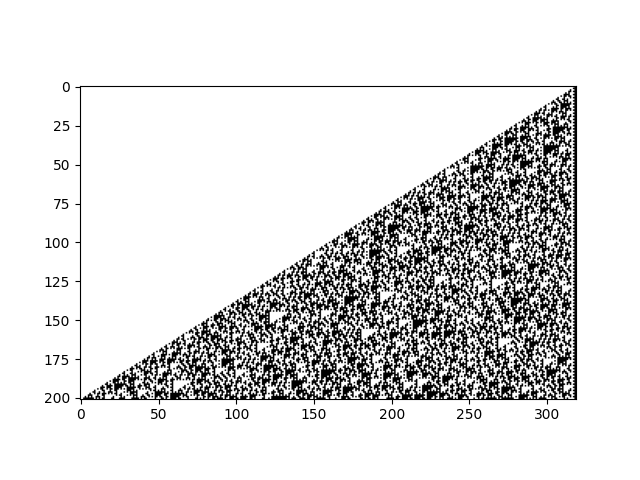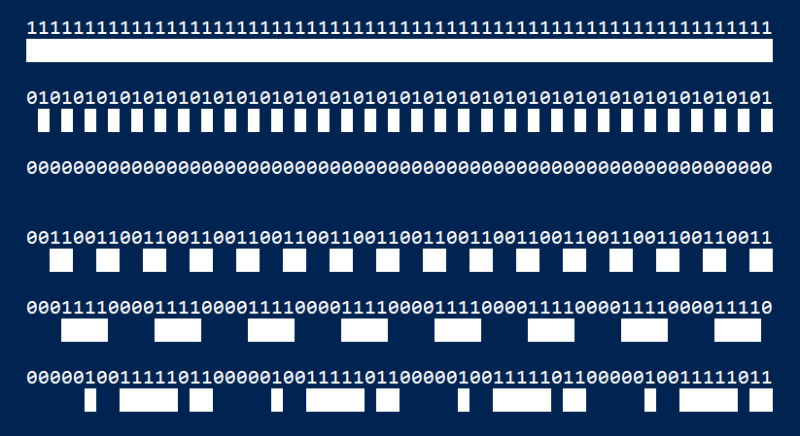# Chaotic image out of regular slices

Yesterday I wrote about the bits in powers of 3. That post had a low-resolution image, which has its advantages, but here’s a higher resolution image that also has its advantages.The image looks chaotic. I say this in the colloquial sense, not in the technical sense as in period three implies chaos. I just mean that the image looks noisy.

And yet every vertical slice of this image is periodic! The last n bits of each row depend only on the last n bits of its predecessor, and there are only 2n possible last bits. Some pattern of last n bits must reappear eventually, and when it does, the pattern will repeat from that point on.

To make this easier to see, imagine rotating the image above counterclockwise by 90 degrees. The rows in the image below correspond to the columns in the image above. I’ll print both the bit values and an on/off visualization as in the previous couple posts.Here’s the code that produced the image.

```for k in range(6):
bits = [(3**i & 2**k) >> k for i in range(64)]
for bit in bits:
print(bit, end='')
print()
for bit in bits:
print(chr(0x2588) if bit else ' ', end='')
print("\n")
```

The array `bits` contains the first 64 bits in column number k, numbering columns from the right. That is, it contains the kth least significant bit of the first 64 powers of 3.

Now when I see a repeating pattern of digits, I think of decimal representations of rational numbers. If we put “0.” in front of the bit sequences above and think of them fractions, is there a pattern to these fractions?

In base two we have

```    0.11111111... = 1
0.01010101... = 1/3
0.00000000... = 0
0.00110011... = 1/5
0.00011110... = 2/17
```

Can you detect a pattern in the rational numbers on the right side?